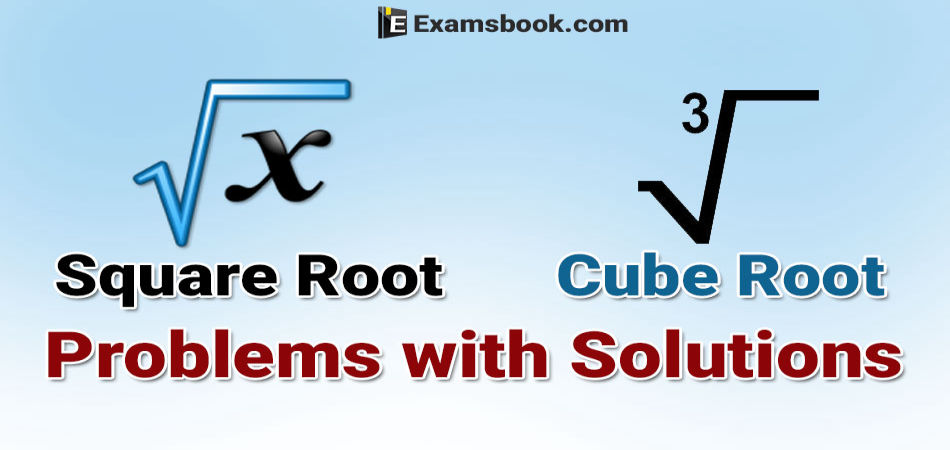• Save

# Square Root and Cube Root Problems with Solutions for SSC and Bank Exams

Vikram Singh7 months ago 5.8K ViewsDo you face difficulty to solve square root and cube root problems in the competitive exams or in your preparation? Here in this blog, I am sharing square root and cube root problems solutions with different examples.

You can learn easily how to solve your square root and cube root problems to score good marks with the help of these questions. First try to solve yourself square root and cube root problems before taking help examples.

Visit here for more practice square root and cube root questions with answers. If you want to know more problems, can visit Cube Problems with Solutions for better performance.

## Square Root and Cube Root Problems Solutions for Competitive Exams

$$Q.1. \ \sqrt { 110 {1\over4}} \ =?$$

(A)10.25               (B) 10.25               (C) 11.5                 (D) 19.5

Solution

$$\sqrt {110 {1\over4}}\ =\sqrt { {441\over4}}\ =\sqrt { {441\over \sqrt { 4} \ }}\ = {21\over2}=10.5$$

$$Q.2. \ \sqrt { {25\over81}-{1\over9}} \ =?$$

$$(A) \ {2\over3} \ (B) \ {4\over9} \ (C) \ {16\over81} \ (D)\ {25\over81} \$$

Solution

$$\sqrt { {25\over81}-{1\over9}}\ =\sqrt { {25-9\over81}}\ =\sqrt { {16\over 81 }}\ = {\sqrt { 16} \ \over \sqrt {81 } \ }={4\over9}$$

Q.3. How many tow-digit numbers satisfy this property: The last digit (unit’s digit) of the square of the tow-digit number is 8 ?

(A) 1                       (B) 2                       (C) 3                       (D) None of these

Solution

A number ending in 8 can never be a perfect square.

Q.4. What is the square root of 0.16?

(A) 0.004                              (B) 0.04                 (C) 0.4                   (D) 4

Solution

$$\sqrt { 0.16}\ =\sqrt { {16\over 100 }}\ = {\sqrt { 16} \ \over \sqrt {100 } \ }={4\over10}=0.4$$

$$Q.5. The \ value \ of \sqrt {0.000441 } \ is :$$

(A) 0.00021                          (B) 0.0021                            (C) 0.021                               (D) 0.21

Solution

$$\sqrt { 0.000441}\ =\sqrt { {441\over 10^6 }}\ = {\sqrt { 441} \ \over \sqrt {10^6 } \ }={21\over10^3}={21\over1000}=.021.$$

Q.6. A group of students decided to collect as many paise from each member of the group as is the number of members. If the total collection amounts to Rs. 59.29, the number of members in the group is:

(A) 57                    (B) 67                     (C) 77                     (D) 87

Solution

Money collected = (59.29×100) paise = 5929 paise. $$∴ Number \ of \ members = \sqrt {5929 } \ =77$$

Q.7. The cube root of .000216 is :

(A) .6                     (B) .06                   (C) .006                (D) None of these

Solution

$$(.000216)^{1/3}=\left({216\over 10^6} \right)=\left({6×6×6\over 10^2×10^2×10^2} \right)^{1/3}={6\over{10}^2}={6\over100}=.06$$

$$Q.8. \ ^3 \sqrt { 4{12\over125}} \ =?$$

$$(A)\ 1{2\over5} \ (B)\ 1{3\over5} \ (C)\ 1{4\over5} \ (D)\ 2{2\over5}$$

Solution

$$^3\sqrt {4{12\over125} } \ = \ ^3\sqrt {{512\over125} } \ =\left({8×8×8\over 5×5×5} \right)^{1/3}={8\over5}= 1{3\over5}$$

Q.9. The largest four-digit number which is perfect cube, is:

(A) 8000                (B) 9261                (C)9999                 (D) None of these

Solution

Clearly, 9261 is a perfect cube satisfying the given property.

Q.10. By what least number 675 be multiplied to obtain a number which is a perfect cube?

(A) 5                       (B) 6                       (C) 7                       (D) 8

Solution

675 = 5×5×3×3×3

To make it a a perfect cube, it must be multiplied by 5.

Q.11. What is the smallest number by which 3600 be divided to make it a perfect cube?

(A) 9                       (B) 50                     (C) 300                  (D) 450

Solution

3600 =23×52×32×2.

To make it a perfect cube, it must be divided by 52×32×2 i.e, 450.

I hope these solutions are helpful for your competitive exams. If you have any query regarding square root and cube root problems solutions, you ask me in the comment section.

All the best!